Next: FILTERS IN PARALLEL Up: Spectrum and phase Previous: Phase of a rational

# ROBINSON'S ENERGY-DELAY THEOREM

Here we will see that a minimum-phase filter

has less energy delay than any other one-sided filter with the same spectrum.  More precisely, the energy summed from zero to any time t for the minimum-phase wavelet is greater than or equal to that of any other wavelet with the same spectrum.

Here is how I prove Robinson's energy-delay theorem: compare two wavelets,and,that are identical except for one zero, which is outside the unit circle forand inside for.We can write this as(38) (39)
where b is bigger than s, and F is arbitrary but of degree n. Proving the theorem for complex-valued b and s is left as an exercise. Notice that the spectrum of b+sZ is the same as that of s+bZ. Next, tabulate the terms in question.

 t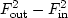bf0 sf01 bf1 + sf0 sf1 + bf0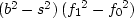k bfk + sfk -1 sfk + bfk -1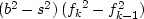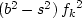n + 1 sfn bfn (b2 - s2) (-fn2)

The difference, which is given in the right-hand column, is always positive. An example of the result is shown in Figure 20.

 robinson Figure 20 Total energy  percentage versus time. x' for minimum-phase wavelet. o' for nonminimum phase.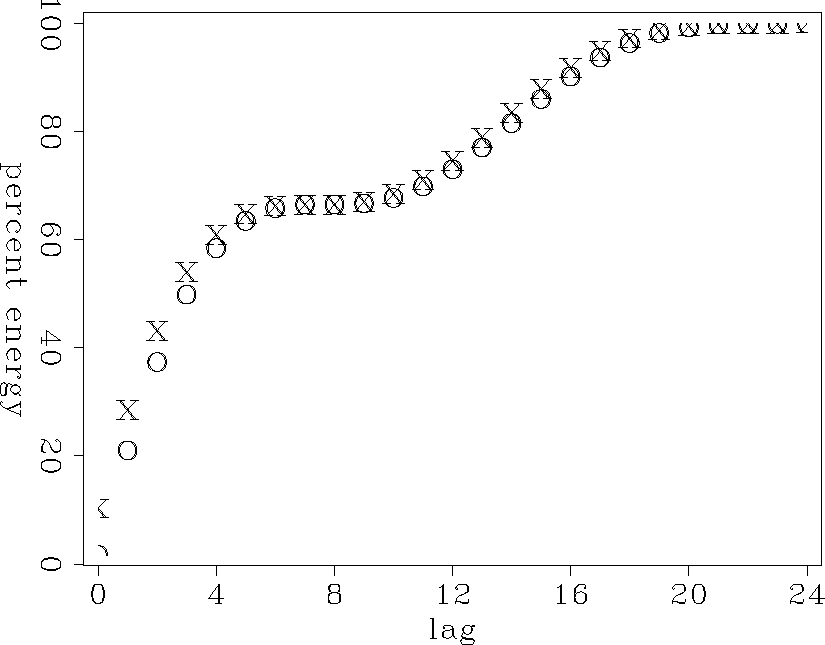Notice that (s+bZ)/(b+sZ) is an all-pass filter. Multiplying by an all-pass filter does not change the amplitude spectrum but instead introduces a zero and a pole. The pole could cancel a preexisting zero, however. To sum up, multiplying by a causal/anticausal all-pass filter can move zeros inside/outside the unit circle. Each time we eliminate a zero inside the unit circle, we cause the energy of the filter to come out earlier. Eventually we run out of zeros inside the unit circle, and the energy comes out as early as possible.

## EXERCISES:

1. Repeat the proof of Robinson's minimum-energy-delay theorem for complex-valued b, s, and fk. (HINT: Doesor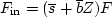?)Next: FILTERS IN PARALLEL Up: Spectrum and phase Previous: Phase of a rational
Stanford Exploration Project
10/21/1998